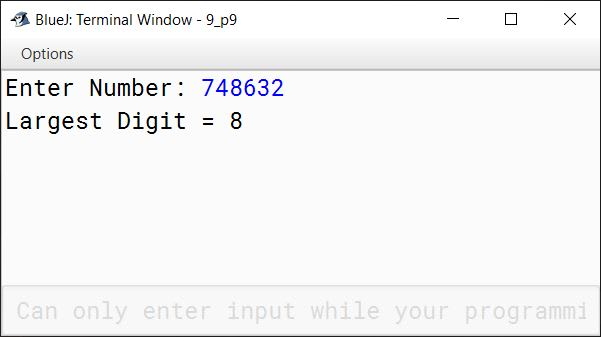# Solved Sample Paper 3

## Section A

#### Question 1

(a) What are the differences between Procedural Programming and Object-Oriented Programming?

Procedural ProgrammingObject-Oriented Programming
Follows a top-down approachFollows a bottom-up approach
Instruction OrientedData Oriented
The abstraction is at procedure (function) level.The abstraction is at object (class) level.
The sequence of events in a large program is divided into functions.Entire program is divided into objects.
Interaction with program is via direct function calls.Interaction with program is via functions defined in the class only.
Real world is represented by 'procedures' operating on data.Real world is represented by objects and the operations that can be performed on these objects.
Data and functions are separate.Data and functions are encapsulated into a single unit.
Data security is less as it contains lesser features to protect the data.It is more secure as one of its primary features include data hiding.
A function can access any other function's data by calling that function.Only the data whose access has been granted can be accessed by another function.
Limited and difficult code reusability.Versatile and easy code reusability.
Code is difficult to modify, extend and maintain.Code is easy to modify, extend and maintain.
Some examples of Procedural Programming languages are C, COBOL, Pascal.Some examples of Object Oriented languages are C++, Java, C#.

(b) Arrange in descending order of byte size the following values:

'c', 94.245, true, 50

94.245, 50, 'c', true

##### Explanation
1. 94.245 is a double and its size is 8 bytes.
2. 50 is an int and its size is 4 bytes.
3. 'c' is a char and its size is 2 bytes.
4. true is a boolean and its size is 1 byte.

(c) Name the package which contains:

1. Scanner class
2. Wrapper classes

1. java.util
2. java.lang

(d) What is meant by Token? Name all the tokens which are used in Java.

A token is the smallest element of a program that is meaningful to the compiler. The different types of tokens in Java are:

1. Identifiers
2. Literals
3. Operators
4. Separators
5. Keywords

(e) Write two differences between Implicit and Explicit conversion.

Implicit ConversionExplicit Conversion
Implicit conversions are automatically performed by the Java compiler.Explicit conversions are performed by the programmer using the cast operator.
Example:
long a = 98453670116L;
long result;
int b = 100;
result = a + b;
Example:
int a = 10;
double b = 25.5;
float c = (float)(a + b);

#### Question 2

(a) Name two jump statements and their use.

1. break statement — It is used to jump out of a switch statement or a loop.
2. continue statement — It is used to skip the current iteration of the loop and start the next iteration.

(b) Write two differences between System.out.print() and System.out.println()

System.out.print()System.out.println()
It prints data to the console but the cursor remains at the end of the data in the same line.It prints data to the console and places the cursor in the next line.
Next printing takes place from the same line.Next printing takes place from next line.

(c) Name the function which:

1. Returns the index of the first occurrence of a character in a string.
2. Returns the largest integer less than or equal to the given number as a double value.

1. indexOf()
2. Math.floor()

(d) Explain the difference between actual and formal parameters.

The parameters that appear in the method definition are called formal or dummy parameters whereas the parameters that appear in the method call are called actual parameters.

(e) What is the purpose of 'new' operator?

The purpose of new operator is to instantiate an object of the class by dynamically allocating memory for it.

#### Question 3

(a) Write a Java expression for the following:

$\sqrt{m(ah+\dfrac{v^2}{2})}$

Math.sqrt(m * (a * h + v * v / 2))

(b) Evaluate the following if the value of x = 20 and y = 20 and z = 10

z *= ++x * (y--) - y

z *= ++x * (y--) - y
⇒ z = z * (++x * (y--) - y)
⇒ z = 10 * (21 * (20) - 19)
⇒ z = 10 * (420 - 19)
⇒ z = 10 * 401
⇒ z = 4010

(c) Give the output of the following program segment:

int x[] = {4, 3, 7, 8, 9, 10};
int p = x.length;
int q = x + x * x;
System.out.println("p=" + p);
System.out.println("q=" + q);

##### Output
p=6
q=37

##### Explanation
1. x.length gives the number of elements in the array which in this case is 6
2. x + x * x ⇒ 7 + 10 * 3 ⇒ 7 + 30 ⇒ 37

(d) Convert the following if else if construct into switch case:

if (a == 10)
System.out.println("TEN");
else if (a == 50)
System.out.println("FIFTY");
else if (a == 100)
System.out.println("HUNDRED");
else
System.out.println("UNKNOWN");

switch (a) {
case 10:
System.out.println("TEN");
break;

case 50:
System.out.println("FIFTY");
break;

case 100:
System.out.println("HUNDRED");
break;

default:
System.out.println("UNKNOWN");
}

(e) Rewrite the following program segment using for loop:

int n = 152, s = 0;
while (n != 0) {
int d = n % 10;
s = s + d;
n = n / 10;
}

int s = 0;
for (int n = 152; n != 0; n = n / 10) {
int d = n % 10;
s = s + d;
}

(f) Rewrite the following program segment using ternary operator:

if (m == 0)
System.out.println("Hello");
else
System.out.println("Good Day");

System.out.println(m == 0 ? "Hello" : "Good Day");

(g) What is a fall through? Give an example.

break statement at the end of case is optional. Omitting break leads to program execution continuing into the next case and onwards till a break statement is encountered or end of switch is reached. This is termed as fall through. For example, consider the below program:

public class FallThroughExample {
public static void main(String args[]) {
int number = 1;
switch(number) {
case 0:
System.out.println("Value of number is zero");
case 1:
System.out.println("Value of number is one");
case 2:
System.out.println("Value of number is two");
default:
System.out.println("Value of number is greater than two");
}
System.out.println("End of switch");
}
}

Its output when executed will be:

Value of number is one
Value of number is two
Value of number is greater than two
End of switch


(h) Give the output of the following program segment and also mention the number of times the loop is executed:

int x, y;
for (x = 9, y = 4; x <= 45; x+=9) {
if (x % y == 0)
break;
}
System.out.println(x);

##### Output
36


Loop executes 4 times

##### Explanation

Below table shows the dry run of the loop:

xyRemarks
941st Iteration
1842nd Iteration
2743rd Iteration
3644th Iteration. As 36 % 4 is 0 so break statement terminates the loop.

(i) Consider the below function:

void strop(String s) {
char a = s.charAt(3);
int b = s.indexOf('M');
String t = s.substring(3,6);
boolean p = s.equals(t);
System.out.println(a + " " + b + " " + t + " " + p);
}

What will be the output for strop("COMPUTER")?

##### Output
P 2 PUT false

##### Explanation
1. s.charAt(3) returns the letter at index 3 of s which is P. This gets assigned to variable a.
2. Index of letter M in s is 2.
3. s.substring(3,6) returns a substring of s starting at index 3 till index 5. So PUT gets assigned to t.
4. As PUT is not equal to the value of s (which is COMPUTER) so p becomes false.

(j) Give the output of the following program segment:

System.out.println("nine:" + 5 + 4);
System.out.println("nine:" + (5 + 4));

##### Output
"nine:54"
"nine:9"

##### Explanation
1. First "nine:" + 5 is evaluated. As one operand of addition operator is string so implicit conversion converts 5 to string and concatenates it with "nine:" and the result is "nine:5". Next "nine:5" + 4 is evaluated and similarly it results in "nine:54".
2. Due to brackets, first (5 + 4) is evaluated. As both operands are numeric so arithmetic addition takes place resulting in 9. Next "nine:" + 9 is evaluated and results in "nine:9".

## Section B

#### Question 4

Define a class named Customer with the following description:

Instance variables /Data members:

String cardName — Name of the card holder.

long cardNo — Card Number.

char cardType — Type of card, 'P' for Platinum, 'G' for Gold, 'S' for Silver.

double purchaseAmount — The amount of purchase done on card.

double cashback — The amount of cashback received.

Member methods:

Customer(String name, long num, char type, double amt) — Parameterised constructor to initialize all data members.

void compute() — To compute the cashback based on purchase amount as follows:

1. For Silver ('S'), 2% of purchase amount
2. For Gold ('G'), 5% of purchase amount
3. For Platinum ('P'), 8% of purchase amount

void display() — To display the details in the below format:

Card Holder Name:
Card Number:
Purchase Amount:
Cashback:

Write a main method to input the card details from the user then create object of the class and call the member methods with the provided details.

import java.util.Scanner;

public class Customer
{
String cardName;
long cardNo;
char cardType;
double purchaseAmount;
double cashback;

public Customer(String name,
long num,
char type,
double amt) {
cardName = name;
cardNo = num;
cardType = type;
purchaseAmount = amt;
cashback = 0;
}

void compute() {
switch (cardType) {
case 'S':
cashback = 2.0 * purchaseAmount / 100.0;
break;

case 'G':
cashback = 5.0 * purchaseAmount / 100.0;
break;

case 'P':
cashback = 8.0 * purchaseAmount / 100.0;
break;

default:
System.out.println("INVALID CARD TYPE");
}
}

void display() {
System.out.println("Card Holder Name: " + cardName);
System.out.println("Card Number: " + cardNo);
System.out.println("Purchase Amount: " + purchaseAmount);
System.out.println("Cashback: " + cashback);
}

public static void main(String args[]) {
Scanner in = new Scanner(System.in);
System.out.println("Enter Card Holder Name:");
String n = in.nextLine();
System.out.println("Enter Card Number:");
long no = in.nextLong();
System.out.println("Enter Card Type:");
char t = in.next().charAt(0);
System.out.println("Enter Purchase Amount:");
double a = in.nextDouble();

Customer obj = new Customer(n, no, t, a);
obj.compute();
obj.display();
}
}
##### Output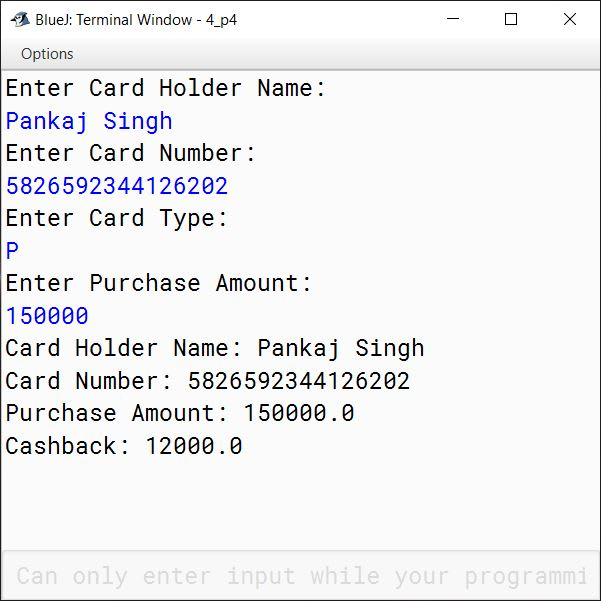#### Question 5

Using switch-case statement write a menu driven program for the following:

1. To display the pattern given below:
A
B C
D E F
G H I J
K L M N O

2. To display the sum of the series given below:
1/1! + 3/3! + 5/5! + ..... + n/n!

import java.util.Scanner;

{
public static void main(String args[]) {
Scanner in = new Scanner(System.in);
System.out.println("Type 1 for Pattern");
System.out.println("Type 2 for Series");
int choice = in.nextInt();
switch (choice) {
case 1:
char ch = 'A';
for (int i = 1; i <= 5; i++) {
for (int j = 1; j <= i; j++) {
System.out.print(ch + " ");
ch++;
}
System.out.println();
}
break;

case 2:
System.out.print("Enter n: ");
int n = in.nextInt();
double s = 0;
for (int i = 1; i <= n; i++) {
double f = 1;
for (int j = 1; j <= i; j++) {
f *= j;
}
double t = i / f;
s += t;
}
System.out.println("Series Sum = " + s);
break;

default:
System.out.println("Incorrect Choice");
}
}
}
##### Output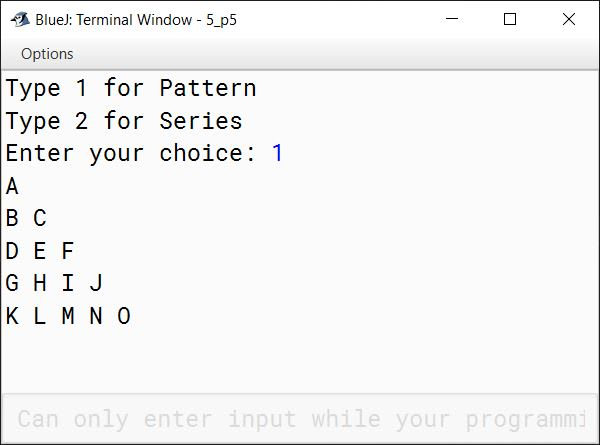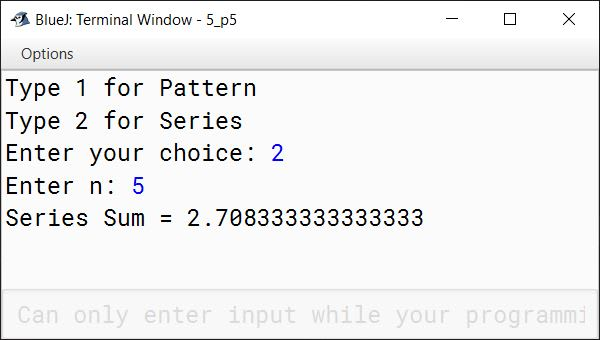#### Question 6

Write a program to input and store roll number and total marks of 20 students. Using Bubble Sort technique generate a merit list. Print the merit list in two columns containing roll number and total marks in the below format:

Roll No.    Total Marks
...                ...
...                ...
...                ...

import java.util.Scanner;

public class MeritList
{
public static void main(String args[]) {
Scanner in = new Scanner(System.in);
int n = 20;
int rollNum[] = new int[n];
int marks[] = new int[n];
for (int i = 0; i < n; i++) {
System.out.print("Enter Roll Number: ");
rollNum[i] = in.nextInt();
System.out.print("Enter marks: ");
marks[i] = in.nextInt();
}

//Bubble Sort
for (int i = 0; i < n - 1; i++) {
for (int j = 0; j < n - i - 1; j++) {
if (marks[j] < marks[j + 1]) {
int t = marks[j];
marks[j] = marks[j+1];
marks[j+1] = t;

t = rollNum[j];
rollNum[j] = rollNum[j+1];
rollNum[j+1] = t;
}
}
}

System.out.println("Roll No.\t\tTotal Marks");
for (int i = 0; i < n; i++) {
System.out.println(rollNum[i] + "\t\t" + marks[i]);
}
}
}
##### Output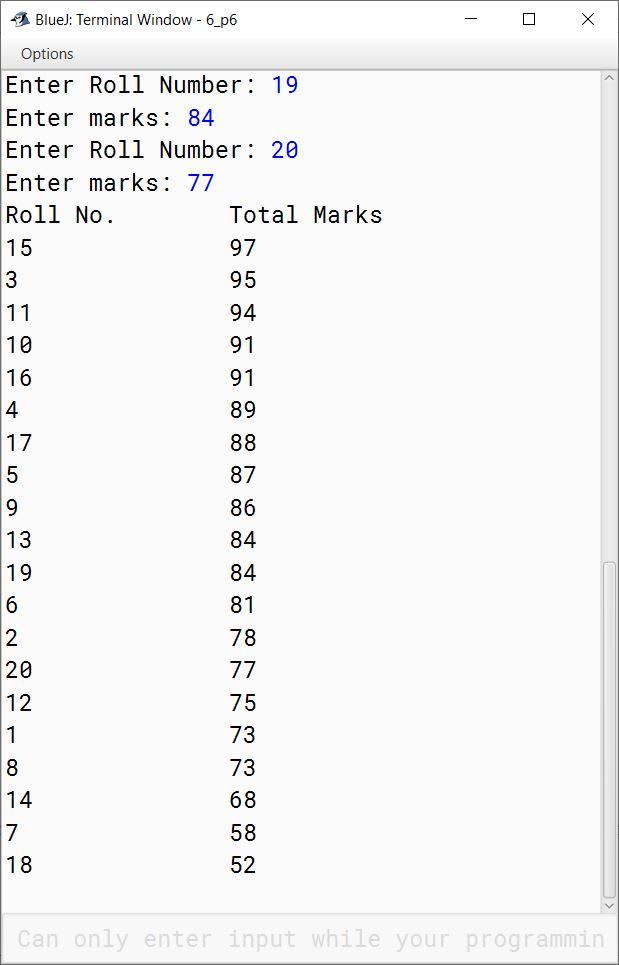#### Question 7

Write a class with the name Area using function overloading that computes the area of a parallelogram, a rhombus and a trapezium.

Formula:

Area of a parallelogram (pg) = base * ht

Area of a rhombus (rh) = (1/2) * d1 * d2
(where, d1 and d2 are the diagonals)

Area of a trapezium (tr) = (1/2) * ( a + b) * h
(where a and b are the parallel sides, h is the perpendicular distance between the parallel sides)

Write a main method to create an object and invoke the above methods.

import java.util.Scanner;

public class Area
{
public double area(double base, double height) {
double a = base * height;
return a;
}

public double area(double c, double d1, double d2) {
double a = c * d1 * d2;
return a;
}

public double area(double c, double a, double b, double h) {
double x = c * (a + b) * h;
return x;
}

public static void main(String args[]) {
Scanner in = new Scanner(System.in);
Area obj = new Area();

System.out.print("Enter base of parallelogram: ");
double base = in.nextDouble();
System.out.print("Enter height of parallelogram: ");
double ht = in.nextDouble();
System.out.println("Area of parallelogram = " + obj.area(base, ht));

System.out.print("Enter first diagonal of rhombus: ");
double d1 = in.nextDouble();
System.out.print("Enter second diagonal of rhombus: ");
double d2 = in.nextDouble();
System.out.println("Area of rhombus = " + obj.area(0.5, d1, d2));

System.out.print("Enter first parallel side of trapezium: ");
double a = in.nextDouble();
System.out.print("Enter second parallel side of trapezium: ");
double b = in.nextDouble();
System.out.print("Enter height of trapezium: ");
double h = in.nextDouble();
System.out.println("Area of trapezium = " + obj.area(0.5, a, b, h));
}
}
##### Output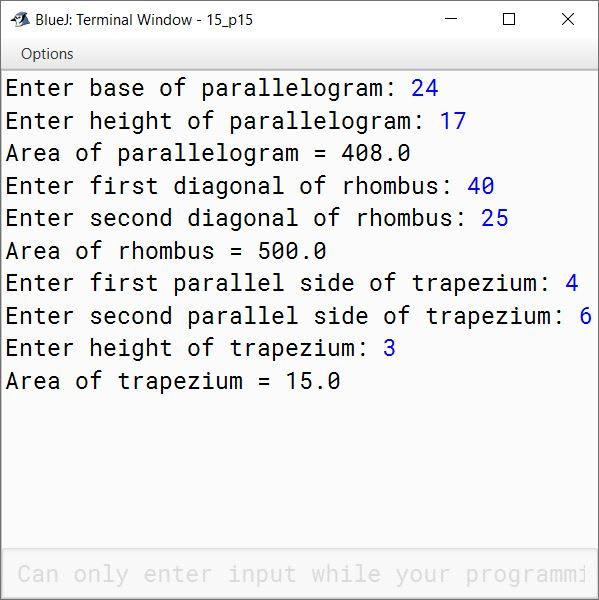#### Question 8

Write a program to print the sum of negative numbers, sum of positive even numbers and sum of positive odd numbers from a list of numbers entered by the user. The list terminates when the user enters a zero.

import java.util.Scanner;

public class KboatNumberSum
{
public static void main(String args[]) {
Scanner in = new Scanner(System.in);
int nSum = 0, eSum = 0, oSum = 0;
System.out.println("Enter Numbers:");
while (true) {
int n = in.nextInt();

if (n == 0) {
break;
}

if (n < 0) {
nSum += n;
}
else if (n % 2 == 0) {
eSum += n;
}
else {
oSum += n;
}
}

System.out.println("Negative No. Sum = " + nSum);
System.out.println("Positive Even No. Sum = " + eSum);
System.out.println("Positive Odd No. Sum = " + oSum);
}
}
##### Output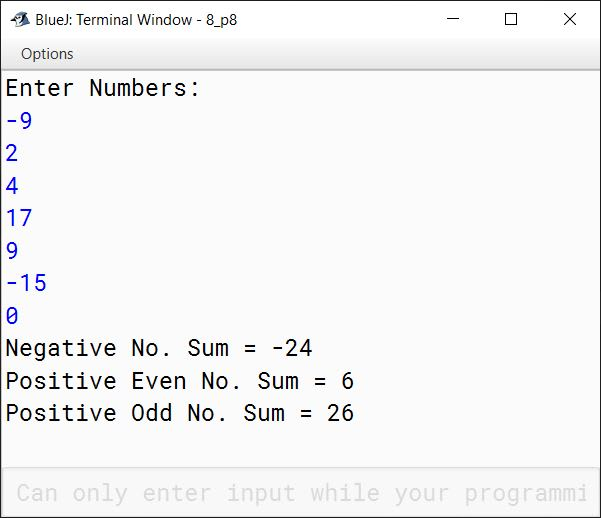#### Question 9

Write a program to take a number from the user as input. Find and print the largest digit of the number.

Example:

Sample Input: 748623
Largest digit: 8

import java.util.Scanner;

public class KboatLargestDigit
{

public static void main(String args[]) {
Scanner in = new Scanner(System.in);
System.out.print("Enter Number: ");
int n = in.nextInt();
int l = -1;
while (n != 0) {
int d = n % 10;
if (d > l)
l = d;
n /= 10;
}
System.out.println("Largest Digit = " + l);
}
}
##### Output